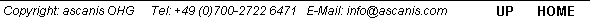# OptLab-SPX   Color Difference Calculations

DIN 6174 (1979) describes the mathematical procedure to calculate the color difference of body colors at low levels of color differences. The procedure is based on the Lab* color space as defined by CIE in 1976. Other norms refer to DIN 6174.

Calculation of color difference uses the L*, a* and b* color values of sample and reference. There is no given illumination, observer or measurement geometry, which means that the parameters must be given with the color difference results. The total color difference is described by the value Delta E*ab , which usually is used as the test value. Additionally it is possible to split up the contributions:

rL*ab               Lightness contribution
rC*ab              Chroma contribution
rH*ab              Hue contribution

Additionally, the differences r a* and r b* are given, which represent the differences of sample and reference on the red/green scale and the yellow/blue scale. All these value serve to define the type or direction of color difference between sample and reference.

OptLab-SPX calculates the overall color difference and the five contributions. As a reference, either a set of Lab* values or a spectrum of a reference can be given.

As with other parameters, the OptLab-SPX parameter file defines the calculation parameter, which is presented in the Calculation selection list. For each parameter either a set of Lab* values or a reference spectrum can be given. Additionally, the output heading, number format and illuminant/observer/wavelength range can e defined.

Further color difference options

Additionally the software offers the calculation of CIEDE2000 color difference values as defined by the recent norm DIN EN ISO 11664-6:2014. In contrat to older norms given above, this norm uses data of the 360-830 nm spectral range, see also Color measurement. The procedure uses sophisticated mathematics with weighting of the various color difference contributions. Thus, CIEDE2000 color difference currently represents the most accepted option to express especially small color differences.

Alternatively, also CMC (1:1, 2:1) color differences can be calculated.